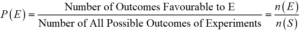Friday, October 22, 2021
Home > CBSE Class 10 > Probability CBSE NCERT Notes Class 10 Maths Chapter 15 PDF

# Probability CBSE NCERT Notes Class 10 Maths Chapter 15 PDFHi students, Welcome to AMBiPi (Amans Maths Blogs). In this article, you will get Probability CBSE NCERT Notes Class 10 Maths Chapter 15 PDF. You can download this PDF and save it in your mobile device or laptop etc.

# Probability CBSE NCERT Notes Class 10 Maths Chapter 15 PDF

## Basic Terms Used in Probability

### Experiments

An operation which results in some well defined outcomes is called an experiment.

### Sample Space

The set of all possible outcomes of a random experiment is called sample space.

### Events

A subset of the sample space of a random experiment is called an event.

### Simple Events/Elementary Events

An event having only one outcome of the experiment is called an elementary event.

The sum of the probabilities of all the elementary events of an experiment is 1.

### Compound Events

An event having more than one outcome of the experiment is called compound event.

### Impossible Events

The probability of an event which is impossible to occur is 0. Such an event is called an impossible event. If P(E) = 0 ⇒ E is an impossible event.

### Sure Events

The probability of an event which is sure (or certain) to occur is 1. Such an event is called a sure event or a certain event. If P(E) = 1 ⇒ E is a sure event.

### Complementary Events

An event which occurs only when event E does not occur is called complementary event of E and it is denoted by E.

## Probability Definition

Probability theory is that branch of mathematics in which we numerically study the chances of occurrence of events and it is defined as## Probability Results

1. 0 ≤ P(E) ≤ 1

2. P(E) ≥ 0 and P(E) ≤ 1

3. P(E) + P(E) = 1 ⇒ P(E) = 1 – P(E)

### Class 10 Maths Chapter 15 Examples 1:

Find the probability of getting a head when a coin is tossed once. Also find the probability of getting a tail.

S = {H, T} ⇒ n(S) = 2 and E = {T} ⇒ n(E) = 1.

Thus, required probability is P(E) = n(E)/n(S) = 1/2

### Class 10 Maths Chapter 15 Examples 2:

One card is drawn from a well-shuffled deck of 52 cards. Calculate the probability that the card will be an ace.

Number of outcomes Favorable to E = 4 and

Number of Possible Outcomes = 52

Thus, P(E) = 4/52 = 1/13

Click below to get CBSE Class 10 Maths Chapter wise Revision Notes PDF.

Chapter NoChapter name
Chapter 1 :Real Numbers CBSE Class 10 Maths Revision Notes
Chapter 2 :Polynomials CBSE Class 10 Maths Revision Notes
Chapter 3 :Pair of Linear Equations in Two Variables CBSE Class 10 Maths Revision Notes
Chapter 4 :Quadratic Equations CBSE Class 10 Maths Revision Notes
Chapter 5 :Arithmetic Progression CBSE Class 10 Maths Revision Notes
Chapter 6 :Triangles CBSE Class 10 Maths Revision Notes
Chapter 7 :Coordinate Geometry CBSE Class 10 Maths Revision Notes
Chapter 8 :Introduction to Trigonometry CBSE Class 10 Maths Revision Notes
Chapter 9 :Some Applications of Trigonometry CBSE Class 10 Maths Revision Notes
Chapter 10 :Circles CBSE Class 10 Maths Revision Notes
Chapter 11 :Constructions CBSE Class 10 Maths Revision Notes
Chapter 12 :Area Related to Circles CBSE Class 10 Maths Revision Notes
Chapter 13 :Surface Areas & Volumes CBSE Class 10 Maths Revision Notes
Chapter 14 :Statistics CBSE Class 10 Maths Revision Notes
Chapter 15 :Probability CBSE Class 10 Maths Revision Notes
AMBiPi
error: Content is protected !!